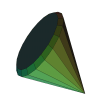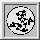URL: http://www.qhull.org

QhullQhull computes convex hulls, Delaunay triangulations, halfspace intersections about a point, Voronoi diagrams, furthest-site Delaunay triangulations, and furthest-site Voronoi diagrams. It runs in 2-d, 3-d, 4-d, and higher dimensions. It implements the Quickhull algorithm for computing the convex hull. Qhull handles roundoff errors from floating point arithmetic. It computes volumes, surface areas, and approximations to the convex hull.

Qhull does not support constrained Delaunay triangulations, triangulation of non-convex surfaces, mesh generation of non-convex objects, or medium-sized inputs in 9-D and higher.

Introduction

Qhull Documentation and Support

Related URLs

FAQs and Newsgroups

The program includes options for input transformations, randomization, tracing, multiple output formats, and execution statistics. The program can be called from within your application.

You can view the results in 2-d, 3-d and 4-d with Geomview. An alternative is VTK.

Barber, C.B., Dobkin, D.P., and Huhdanpaa, H.T., "The Quickhull algorithm for convex hulls," ACM Trans. on Mathematical Software, Dec 1996. http://www.acm.org;

Abstract:

The convex hull of a set of points is the smallest convex set that contains the points. This article presents a practical convex hull algorithm that combines the two-dimensional Quickhull Algorithm with the general dimension Beneath-Beyond Algorithm. It is similar to the randomized, incremental algorithms for convex hull and Delaunay triangulation. We provide empirical evidence that the algorithm runs faster when the input contains non-extreme points, and that it uses less memory.

Computational geometry algorithms have traditionally assumed that input sets are well behaved. When an algorithm is implemented with floating point arithmetic, this assumption can lead to serious errors. We briefly describe a solution to this problem when computing the convex hull in two, three, or four dimensions. The output is a set of "thick" facets that contain all possible exact convex hulls of the input. A variation is effective in five or more dimensions.

Up: Past Software Projects of the Geometry Center
URL: http://www.qhull.orgThe Geometry Center Home Page